# Does Voltage Change Across a Capacitor?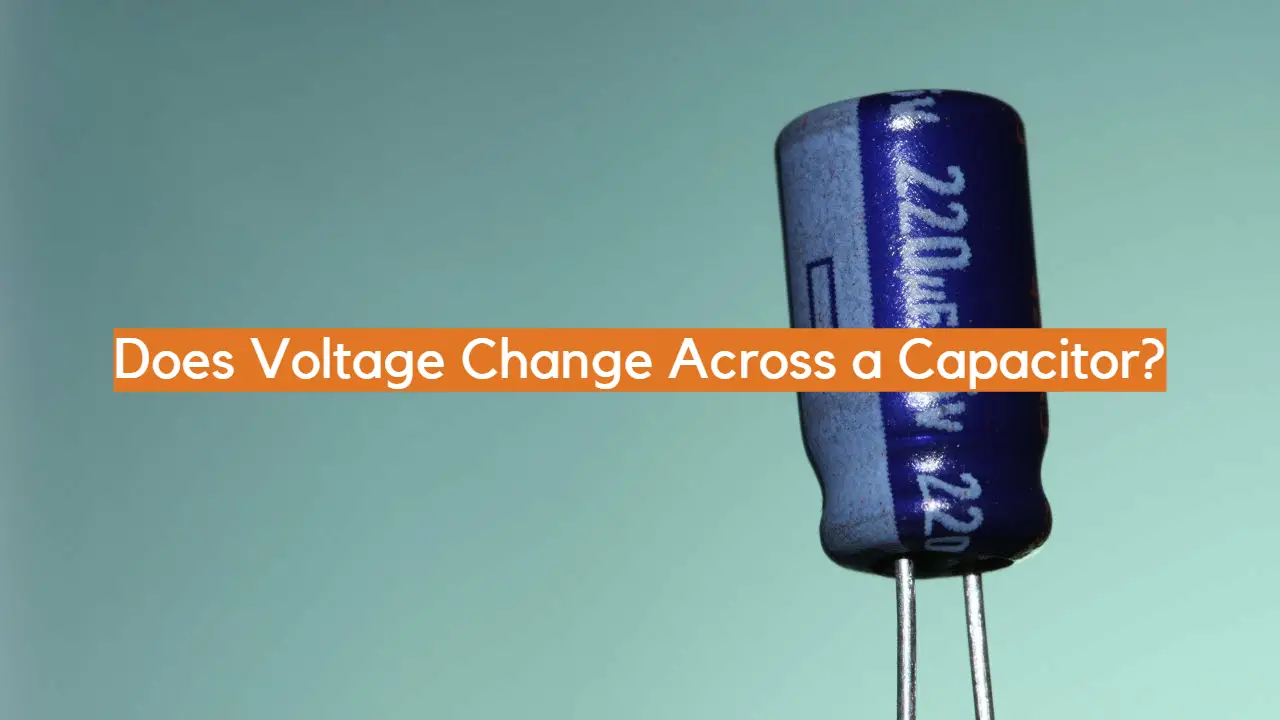In the world of electronic circuits and devices, capacitors are indispensable components that play a vital role in various applications. These passive electronic components have the unique ability to store and release electrical energy in the form of an electric field between their plates.

Understanding the behavior of capacitors is essential for engineers, hobbyists, and enthusiasts alike to design and analyze circuits effectively. One fundamental question that often arises in the study of capacitors is whether voltage changes across them.

In this article, we will explore the intricate relationship between voltage and capacitors, shedding light on their behavior during charging, discharging, and steady-state conditions. We will delve into the charging process, where a capacitor increases its voltage when connected to a voltage source, and examine the factors influencing this behavior, such as capacitance and time constants.

Additionally, we will uncover the process of discharging, where a capacitor releases its stored charge, leading to a decrease in voltage. Moreover, we will investigate how capacitors behave in steady-state situations, revealing whether the voltage remains constant once the capacitor is fully charged or discharged.

By the end of this article, readers will gain a comprehensive understanding of whether voltage changes across capacitors and how this phenomenon impacts their functionality in electronic circuits.

## Understanding Capacitors

A capacitor is a passive electronic component that stores electrical energy by collecting electric charge on two electrodes separated by a dielectric material .

Capacitance is the measure of how much electrical charge a capacitor can store for a given voltage. The capacitance value of a capacitor is measured in Farads (F) and is defined as the ratio of the charge stored per unit voltage across the capacitor.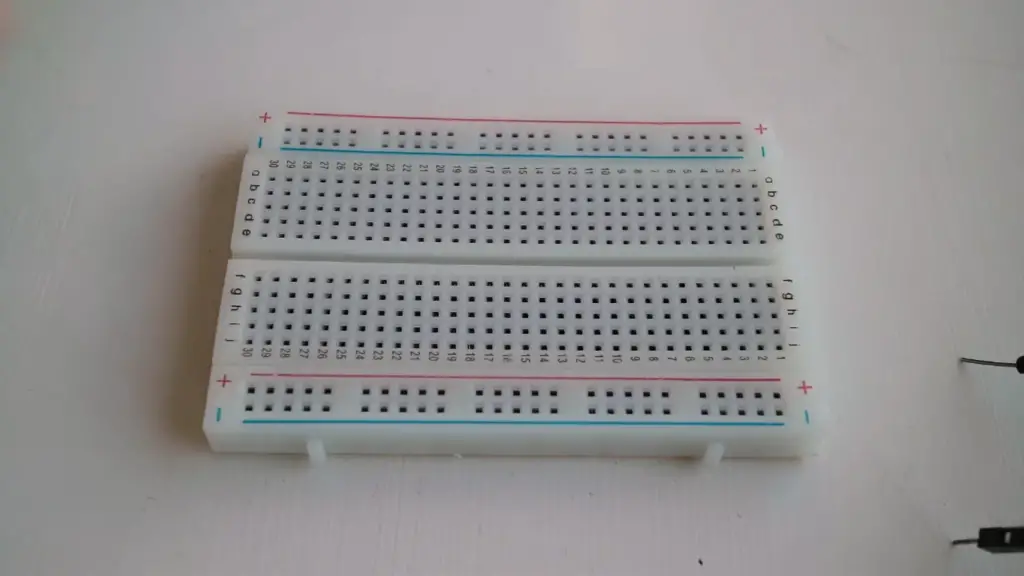When a voltage is applied, the electrons are attracted to either side of the capacitor, creating a charge separation. The resulting electric field stores the energy in the form of potential energy. Capacitors can store electrical energy like a battery, but they release it more rapidly.

In order to understand the voltage across a capacitor, it is essential to understand the working mechanism of a capacitor. A capacitor consists of two conductive plates separated by an insulating dielectric material. When a voltage is applied across the plates, an electric field is created, resulting in an accumulation of charges on each plate.

The charge on the capacitor stores up energy, and the capacitor is said to be charged. The amount of charge stored and the voltage across the capacitor is determined by the capacitance, which is a measure of the amount of charge that the capacitor can store.

Various factors can affect the capacitance of a capacitor, such as the distance between the plates, the area of the plates, and the dielectric constant of the material separating the plates.

### The Charging Process

When a capacitor is initially connected to a voltage source, such as a battery, it starts to charge. Electrons flow from the negative terminal of the voltage source, through the capacitor, to the positive terminal. During this charging process, the voltage across the capacitor gradually increases as more charge accumulates on its plates.

However, it is crucial to note that the voltage across the capacitor does not change instantly but rather follows an exponential curve until it reaches its maximum value, determined by the voltage of the source.

### Steady State: A Misunderstood Concept

The confusion surrounding whether voltage changes across a capacitor often arises from the concept of “steady state” . In a steady state, the capacitor has fully charged, and the flow of charge ceases. As a result, some may mistakenly believe that the voltage across the capacitor remains constant. However, this is not entirely accurate. While the voltage across the capacitor does not change once it reaches its maximum value during the steady state, it is essential to understand that voltage fluctuations do occur during the charging process, leading to the established steady-state voltage.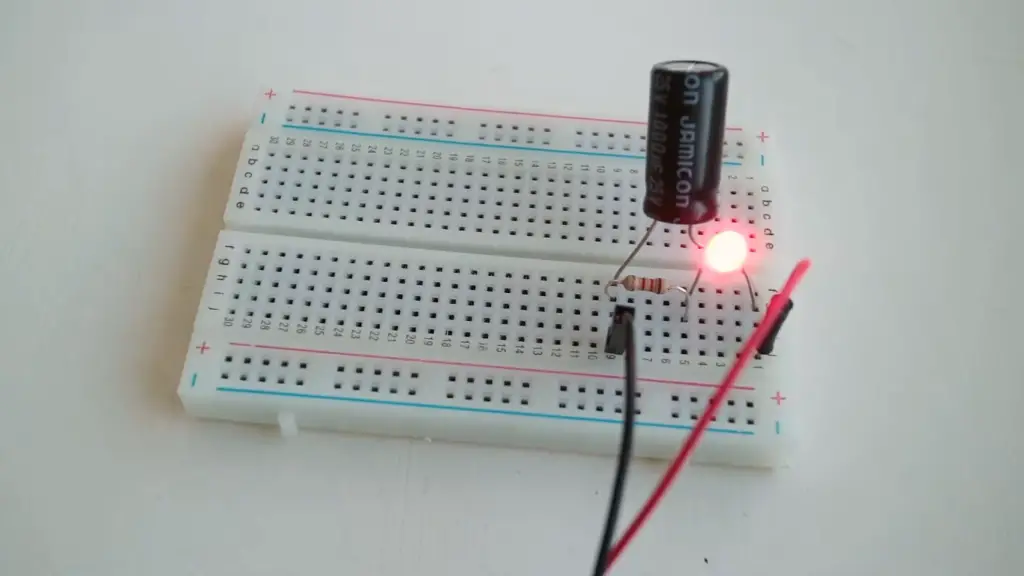### Voltage Changes During Transients

One crucial aspect that further dispels the myth is examining the behavior of capacitors during transient states. Transients refer to the period when a circuit’s input conditions change, causing the system to respond to these changes. During transients, the voltage across a capacitor does experience fluctuations until the system reaches a new equilibrium.

For instance, if a capacitor is connected to a new voltage source with a different potential, the voltage across the capacitor will not immediately adjust to the new value. Instead, it will undergo a transition, gradually reaching the new voltage over time. This transient behavior showcases that the voltage across the capacitor does change under specific circumstances.

## Understanding Voltage Drops of Capacitors and Inductors:

### Capacitor Voltage Drops

A capacitor is a passive two-terminal electronic component that stores electrical energy in the form of an electric field. When a voltage is applied across a capacitor, it charges up, storing energy in the form of an electric field between its two plates. As it charges, the voltage across the capacitor increases until it reaches the same potential as the applied voltage.

However, when the voltage across the capacitor changes, it does not instantaneously follow the voltage change due to its inherent property known as capacitance. Capacitors resist changes in voltage by opposing sudden voltage variations. This opposition to voltage changes leads to the concept of the capacitor voltage drop.

When a sudden increase in voltage is applied to a capacitor, it initially acts as a short circuit, allowing a large current to flow. As the capacitor charges, the current decreases, and the voltage across the capacitor increases gradually. The rate at which the voltage changes depends on the time constant, which is the product of the capacitance (C) and the resistance (R) in the circuit. A higher time constant means the voltage changes more slowly, and vice versa.

Conversely, when the voltage across the capacitor decreases suddenly, it behaves as an open circuit and opposes the sudden decrease in voltage. The capacitor discharges, allowing current to flow, but the voltage across the capacitor decreases slowly due to the time constant.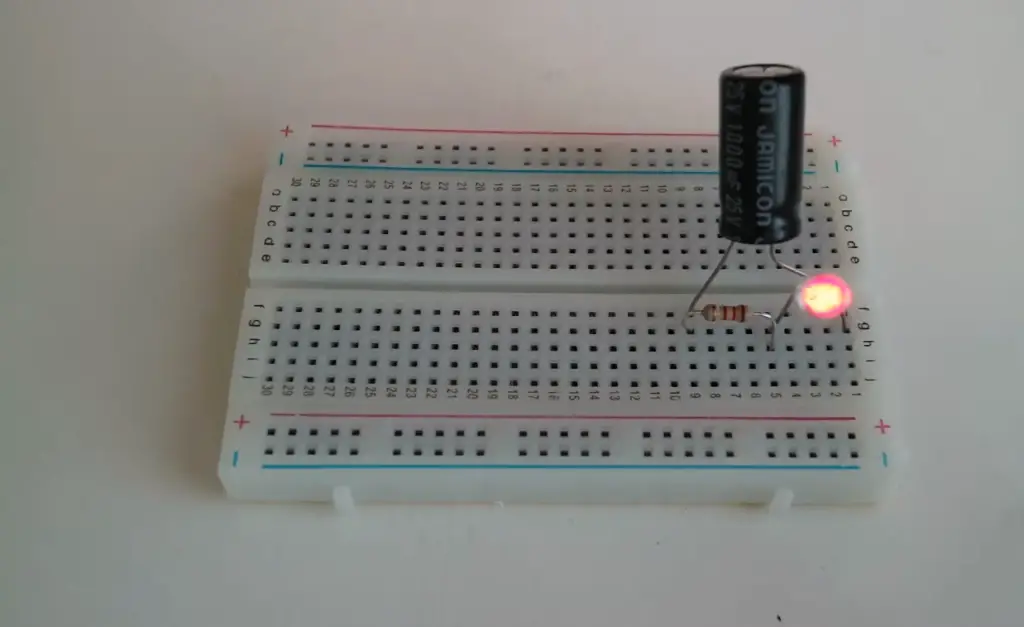### Inductor Voltage Drops

An inductor is another passive two-terminal electronic component that stores energy in the form of a magnetic field when current passes through it. Unlike capacitors, inductors resist changes in current by generating a voltage across their terminals opposing the change. This opposition to current changes leads to the concept of the inductor voltage drop.

When current starts to flow through an inductor, the magnetic field builds up, inducing a back EMF (electromotive force) that opposes the rise of current. As a result, the voltage across the inductor initially increases slowly. The rate at which the voltage changes depends on the inductance (L) of the inductor and the rate of change of current. A higher inductance value means the voltage changes more slowly, and vice versa.

Conversely, when the current through the inductor decreases suddenly, the magnetic field collapses, inducing a voltage that opposes the sudden drop in current. The inductor now acts as a source of energy, pushing current through the circuit to maintain the current flow, and the voltage across the inductor decreases slowly due to the inductor’s inductance.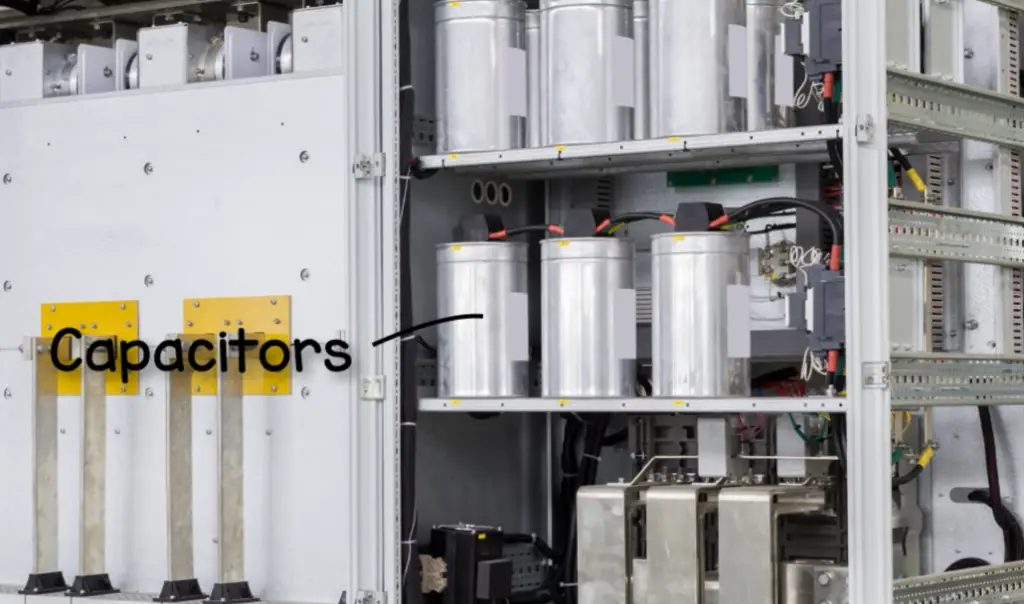### Changes in Current

Both capacitors and inductors respond differently to changes in current due to their inherent properties. Capacitors oppose changes in voltage, and their current response depends on the capacitance and the applied voltage. On the other hand, inductors oppose changes in current, and their voltage response depends on the inductance and the rate of change of current.

In an AC (alternating current) circuit, both capacitors and inductors play vital roles. Capacitors pass AC signals better as the frequency increases because their impedance decreases with frequency . On the other hand, inductors have higher impedance at higher frequencies, making them suitable for filtering low-frequency signals.

Furthermore, when capacitors and inductors are combined in a circuit, they form important building blocks for filters, oscillators, and other signal processing applications. The interaction between these components can lead to interesting circuit behaviors, such as resonance in certain configurations.

## How Does Voltage Change Across a Capacitor:

### Charging a Capacitor

When a capacitor is connected to a voltage source, such as a battery or power supply, and a circuit is closed, the capacitor starts to charge. At the initial moment of connection, the capacitor behaves like an uncharged state, acting as a short circuit with negligible resistance. As a result, current flows freely through the capacitor, and the voltage across it rises.

The rate at which the voltage across the capacitor changes during charging depends on its capacitance (C) and the resistance (R) in the circuit, often referred to as the time constant (τ). The time constant determines how quickly the capacitor reaches its final charged voltage.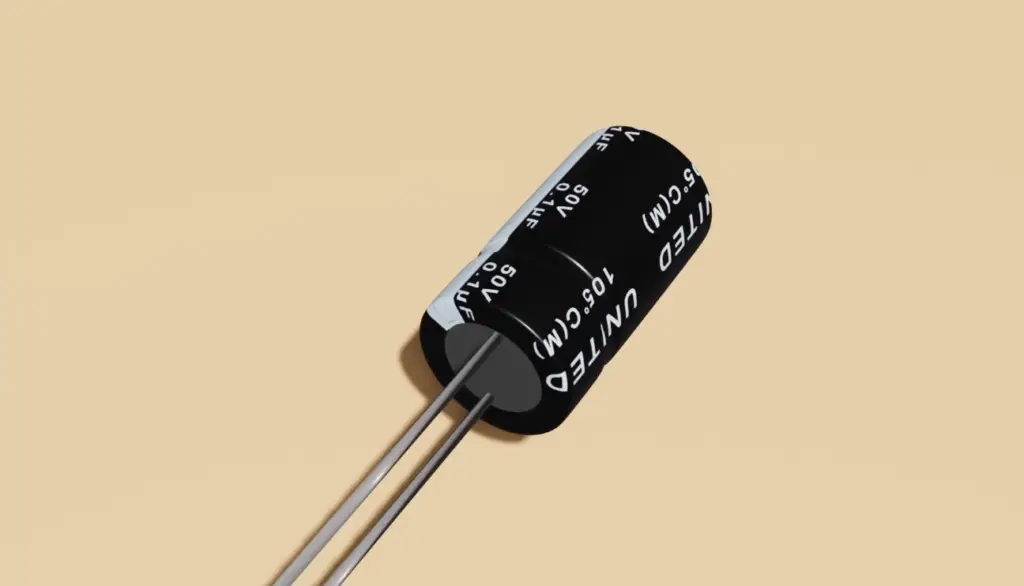Mathematically, the voltage across the charging capacitor (Vc) at any given time (t) can be expressed by the formula:

Vc(t) = Vsource * (1 – e^(-t/τ))

Where:

• Vc(t) is the voltage across the capacitor at time t
• Vsource is the voltage of the applied source
• e is the base of the natural logarithm
• τ (tau) is the time constant, calculated as τ = R * C

As time (t) approaches infinity, the term e^(-t/τ) becomes negligible, and the capacitor charges up to the same voltage as the applied source.

### Discharging a Capacitor

When the voltage source is disconnected from the capacitor or the circuit is opened, the capacitor begins to discharge. At the moment of disconnection, the capacitor behaves like a fully charged state, acting as an open circuit with infinite resistance. Therefore, no current flows through the capacitor.

The voltage across the discharging capacitor decreases gradually as it discharges its stored energy. The rate of voltage change during discharging also depends on the time constant (τ), which is the product of the capacitance (C) and the resistance (R) in the circuit.

Mathematically, the voltage across the discharging capacitor (Vc) at any given time (t) can be expressed by the formula:

Vc(t) = Vinitial * e^(-t/τ)

Where:

• Vc(t) is the voltage across the capacitor at time t during discharging
• Vinitial is the initial voltage across the capacitor at the moment of disconnection (Vsource voltage).
• e is the base of the natural logarithm
• τ (tau) is the time constant, calculated as τ = R * CAs time (t) approaches infinity, the term e^(-t/τ) approaches zero, and the voltage across the capacitor becomes zero, indicating that the capacitor is fully discharged.

### A Capacitor In an AC Circuit

In an alternating current (AC) circuit, the voltage across a capacitor continually oscillates between positive and negative values. As the AC voltage alternates, the capacitor charges and discharges accordingly. The voltage across the capacitor lags the current by 90 degrees in an AC circuit .

The impedance of a capacitor in an AC circuit is given by:

Zc = 1 / (jωC)

Where:

• Zc is the impedance of the capacitor
• j is the imaginary unit (√(-1))
• ω (omega) is the angular frequency of the AC source
• C is the capacitance of the capacitor

## Summing Up – Does Voltage Change Across a Capacitor?

One of the important questions related to capacitors is whether the voltage changes across the capacitor. The answer to this question depends on the time scale over which the change in voltage is being examined. In the short term, the voltage across the capacitor changes as the capacitor charges and discharges. However, in the long term, the voltage across the capacitor will remain constant.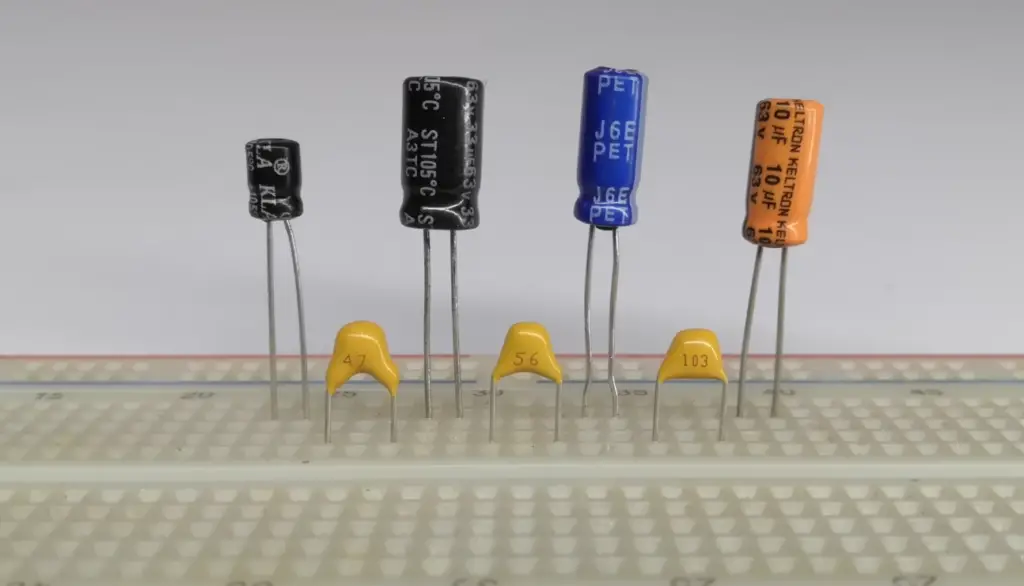When a capacitor is first connected to a voltage source, the voltage across the capacitor is initially zero. As the capacitor begins to charge, the voltage across the capacitor starts to increase until it reaches the same voltage as the voltage source. At this point, the capacitor is fully charged, and the voltage across the capacitor is equal to the voltage of the charging source.

It is essential to note that the voltage across a capacitor reflects the potential difference between its plates, not the potential difference at the points where the capacitor is connected to the circuit. This means that the voltage across the capacitor can be different from the voltage of the power supply.

When the power supply is disconnected, the capacitor starts to discharge, and the voltage across the capacitor decreases. The rate of discharge is dependent on the circuit resistance and the capacitance of the capacitor.

The voltage across a capacitor will keep decreasing until it equals zero. At this point, the capacitor is fully discharged, and the voltage across the capacitor remains zero until another voltage source is connected.

In summary, the voltage across a capacitor does change in the short term when it is charged or discharged, but over the long term, the voltage across the capacitor will remain constant.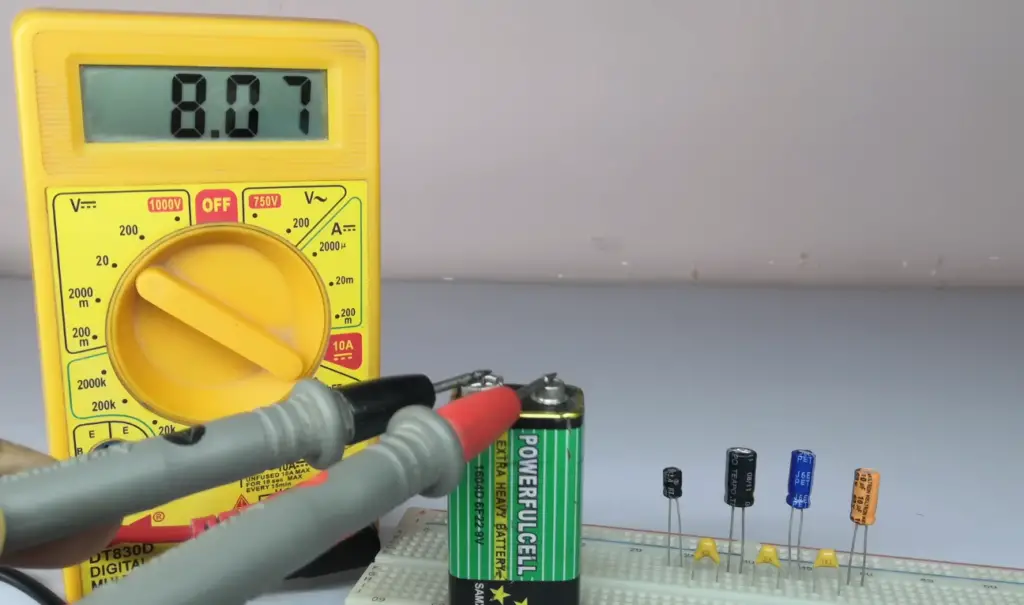## FAQ:

### 1. What happens when capacitors are connected in parallel?

When capacitors are connected in parallel, the positive terminals are connected together, and the negative terminals are also connected together. In this configuration, the voltage across each capacitor is the same, as they share the same potential difference.

However, the total capacitance of the parallel combination is the sum of the individual capacitances:

C_total = C1 + C2 + C3 + …

The equivalent capacitance (C_total) of capacitors in parallel is always greater than any individual capacitance. Connecting capacitors in parallel effectively increases the overall capacitance, allowing the combination to store more charge for a given voltage.

### 2. Is voltage constant across a capacitor?

No, the voltage across a capacitor is not constant in all circumstances. When a capacitor is charging or discharging, the voltage across it changes with time. Initially, when a voltage is applied to a capacitor, the voltage across it increases exponentially during the charging process until it reaches the applied voltage. During discharging, the voltage decreases exponentially until it becomes fully discharged, reaching zero volts.

However, in steady-state conditions or in a DC circuit where the voltage source is constant, and the capacitor has reached its fully charged or fully discharged state, the voltage across the capacitor becomes constant.

### 3. How is voltage affected by a capacitor?

A capacitor affects voltage in a circuit by storing and releasing electrical energy in the form of an electric field. When connected to a voltage source, the capacitor charges up, and its voltage increases until it reaches the same voltage as the applied source. The voltage across the capacitor is directly proportional to the amount of charge it can store, which is determined by its capacitance. A higher capacitance value results in a higher voltage for the same amount of charge stored.

### 4. Does voltage change across capacitors in series?

Yes, in a series configuration, the voltage across capacitors can be different. When capacitors are connected in series, their positive terminals are connected to each other, and their negative terminals are also connected together.

The total voltage across the series combination is the sum of the individual voltages:

V_total = V1 + V2 + V3 + …

The voltage across each capacitor in a series configuration depends on the charge stored on it and its capacitance. Since the capacitors share the same charge, the voltage across each capacitor can be different, depending on their individual capacitance values.

### 5. Does voltage increase or decrease across a capacitor?

The voltage across a capacitor increases when it is charging, i.e., when connected to a voltage source. During the charging process, the voltage across the capacitor rises from zero to the applied voltage.

Conversely, the voltage across a capacitor decreases when it is discharging, i.e., when the circuit is opened or disconnected from the voltage source. During discharging, the voltage across the capacitor decreases gradually until it becomes fully discharged and reaches zero volts.

### 6. Does higher capacitance increase voltage?

A higher capacitance value does not directly increase the voltage across a capacitor. The voltage across a capacitor depends on the applied voltage and the amount of charge it can store, which is determined by its capacitance. A higher capacitance means the capacitor can store more charge for the same applied voltage, but the voltage across the capacitor remains the same as the applied voltage when fully charged.

### 7. Do capacitors store voltage or current?

Capacitors store electrical energy in the form of an electric field between their plates, and they store charge, not voltage or current. When a voltage is applied across a capacitor, it stores charge, which leads to an increase in voltage across the capacitor until it reaches the same voltage as the applied source. Capacitors do not store current, but they can allow current to flow through them depending on the circuit configuration and the changing voltage across the capacitor.

### 8. Does the capacitor lead to voltage or current?

A capacitor leads the current in an AC circuit, but it lags the voltage.

This phase difference between current and voltage is due to the capacitive reactance (Xc) of the capacitor in an AC circuit, given by the formula:

Xc = 1 / (2πfC)

Where:

• Xc is the capacitive reactance
• π is the constant pi (approximately 3.14159)
• f is the frequency of the AC source
• C is the capacitance of the capacitor

### 9. How does the voltage across a capacitor change with time?

The voltage across a capacitor changes with time during the charging and discharging processes. During charging, the voltage across the capacitor increases exponentially until it reaches the applied voltage. The rate of voltage increase depends on the time constant of the charging circuit, which is the product of the capacitance and resistance in the circuit.

During discharging, the voltage across the capacitor decreases exponentially until it becomes fully discharged, reaching zero volts. The rate of voltage decrease depends on the time constant of the discharging circuit, which is again determined by the capacitance and resistance values.

### 10. What happens to voltage when the capacitor is fully charged?

When a capacitor is fully charged, the voltage across it becomes equal to the applied voltage from the voltage source. At this point, the capacitor behaves like an open circuit, and no current flows through it. The voltage remains constant at the applied voltage until the charging process is interrupted or the circuit is opened.

### 11. Can capacitor voltage be negative?

In a DC circuit, the voltage across a capacitor cannot be negative. The voltage across a capacitor during charging and discharging varies between zero and the applied voltage. However, in an AC circuit, the voltage across a capacitor can be negative, as the voltage continually oscillates between positive and negative values.

### 12. Do capacitors divide voltage?

Yes, capacitors can divide voltage in a circuit when they are connected in series. In a series configuration, the total voltage is divided among the individual capacitors based on their capacitance values. The voltage across each capacitor in series depends on the charge stored on it, which is determined by its capacitance.

### 13. Is capacitor voltage AC or DC?

Capacitors can be used in both AC (alternating current) and DC (direct current) circuits. In an AC circuit, the voltage across a capacitor continuously alternates between positive and negative values as the AC source oscillates. In a DC circuit, the voltage across a capacitor initially changes during the charging process but stabilizes once the capacitor is fully charged or discharged.

### 14. Does the voltage on the capacitor equal to the battery?

The voltage across a capacitor can be equal to the voltage of the battery or voltage source to which it is connected during the charging process. However, in steady-state conditions or when the capacitor is fully charged or fully discharged, the voltage across the capacitor remains constant and equal to the applied voltage.

### 15. Why doesn’t voltage change in a parallel circuit?

In a parallel circuit, the voltage remains the same across all elements connected in parallel, including capacitors. This is because all elements in a parallel configuration have the same potential difference or voltage across their terminals. The voltage across the parallel combination is equal to the voltage of the voltage source connected to the circuit.

### 16. How is capacitor voltage different in parallel and series?

In parallel, capacitors have the same voltage across their terminals, while in series, the voltage across each capacitor can be different.

In a parallel configuration, the voltage across each capacitor is equal to the voltage of the voltage source.

In contrast, in a series configuration, the total voltage is divided among the capacitors based on their capacitance values and the amount of charge stored on each capacitor.

### 17. Is voltage always constant in parallel?

Yes, in a parallel circuit, the voltage remains constant across all elements connected in parallel, including capacitors. Each element has the same voltage across its terminals as the voltage of the voltage source. This is one of the defining characteristics of a parallel circuit.

### 18. Does the voltage across the capacitor increases as the capacitor is charging?

Yes, the voltage across the capacitor increases during the charging process. When a capacitor is connected to a voltage source, it charges up, and its voltage increases gradually until it reaches the same voltage as the applied source. The rate of voltage increase depends on the time constant of the charging circuit, which is determined by the capacitance and resistance in the circuit. As the charging process continues, the voltage across the capacitor rises until it becomes fully charged.

### 19. What is a capacitor, and how does it work?

A capacitor is an electrical component that stores electric charge. It consists of two conductive plates separated by an insulating material called the dielectric. When a voltage is applied across the capacitor, positive charge accumulates on one plate, and an equal amount of negative charge accumulates on the other plate, creating an electric field between them.

### 20. What is the concept of “steady state” in relation to capacitors?

In the context of capacitors, “steady state” refers to the point at which the capacitor has fully charged, and the flow of charge ceases. During a steady state, the voltage across the capacitor does not change any further, and the electric field between the plates remains constant.

### 21. Does the voltage across a capacitor remain constant during a steady state?

Yes, during a steady state, the voltage across a capacitor remains constant. However, it is important to understand that the voltage across the capacitor does change during the charging process until it reaches its steady-state value.

### 22. Can the voltage across a capacitor change under certain conditions?

Yes, the voltage across a capacitor can change under specific conditions. During transient states, such as when the circuit’s input conditions change or when a capacitor is connected to a new voltage source, the voltage across the capacitor undergoes fluctuations until the system reaches a new equilibrium.

### 23. How does a capacitor behave in an alternating current (AC) circuit?

In AC circuits, capacitors introduce a new element called “capacitive reactance” (Xc). Capacitive reactance is the opposition that a capacitor presents to the flow of alternating current. As the frequency of the AC signal changes, the capacitive reactance also changes, leading to a varying voltage across the capacitor over time.

### 24. Does the voltage across a capacitor change during the discharging process?

Yes, when a capacitor discharges, the voltage across it changes. During the discharging process, the accumulated charge on the plates flows out, and the voltage across the capacitor decreases. The discharge process follows a similar exponential curve as the charging process but in reverse.

### 25. Why is it important to understand how voltage behaves across capacitors?

Understanding how voltage changes across capacitors are crucial for designing and analyzing electronic circuits. It allows engineers to predict transient behavior during circuit startup or changes and ensure that capacitors function as intended. Additionally, this knowledge is essential for working with AC circuits where capacitive reactance plays a significant role.

### 26. Can capacitors store an infinite amount of charge?

No, capacitors have limits to the amount of charge they can store. The maximum charge a capacitor can hold depends on its capacitance, which is a fixed characteristic determined by its physical properties.

### 27. What are some practical applications of capacitors in electronics?

Capacitors have numerous applications in electronics, including energy storage in power supplies, filtering and smoothing in signal processing, coupling and decoupling in amplifiers, and timing circuits in oscillators, among many others. They are fundamental components in various electronic devices and systems, contributing to their proper functioning and reliability.